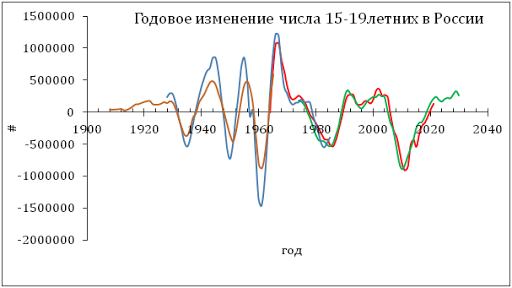## 2/19/12

### Modeling ConocoPhillips share price: annual report

This is an annual report on the performance of our pricing model linking share prices of energy companies with the difference between the headline and core CPI and PPI. In essence, we were trying to use the core CPI (PPI) as an energy independent (dynamic) reference to the index which includes energy. Then the difference might be related to the energy pricing power relative to other goods and services.  This idea has proven to be fruitful for oil (energy) companies and other categories of companies in the S&P 500 index and other consumer price indices.

Our original pricing model states that a share price, for example, that of ConocoPhillips, COP(t), can be approximated by a linear function of the difference between the core CPI, cCPI, and headline CPI:

COP(t) = A + B (cCPI - CPI(t))                                  (1)
where A and B are empirical constants; t is the elapsed time.  This model has proven its predictive power and we have been reporting on its performance since 2009.

In August 2011, we extended the set of defining indices by the consumer price index of energy, eCPI, and the producer price index of crude petroleum, pPPI, together with the overall PPI. Thus, we tested the following models for the period between 2001 and July 2011:

COP(t) = A1 + B1(cCPI - eCPI(t))  (2)
COP(t) = A2 + B2(pPPI - PPI(t))    (3)
Here we use new data through January 2012 retrieved from the BLS website. Figures 1 through 3 compare the original and new predictions for COP. Coefficients in (1) through (3) are given in Figure captions and are the same as in August 2011. The best model, as defined by standard error, for the period between January 2003 and January 2012 is based on the index of energy and core CPI.  (Same model was the best in August.) The accuracy of (1) has decreased since August and reached \$8.31At the same time, model (3) based on the producer price indices is the worst and has failed to predict the amplitude of the largest oscillation in 2008.  It shoul be noted however that the difference between the index of crude oil and PPI perfectly describes the evolution of COP share price since 2009.
One can conclude that the consumer price index of energy has the largest influence on COP share price. Crude oil is well correlated with COP price but  failed to describe variations in the past. It is a good predictor  since 2009.
Figure 1.  The observed COP price and that predicted from the core and headline CPI.  A=75, B=-5.5. Stdev=\$8.31.

Figure 2.  The observed COP price and that predicted from the core CPI and the consumer price index of energy.  A1=58, B1=-0.54. Stdev=\$6.81.
Figure 3.  The observed COP price and that predicted from the overall PPI and the producer price index of crude petroleum (domestic production).  A2=45, B2=-0.3. Stdev=\$9.09.